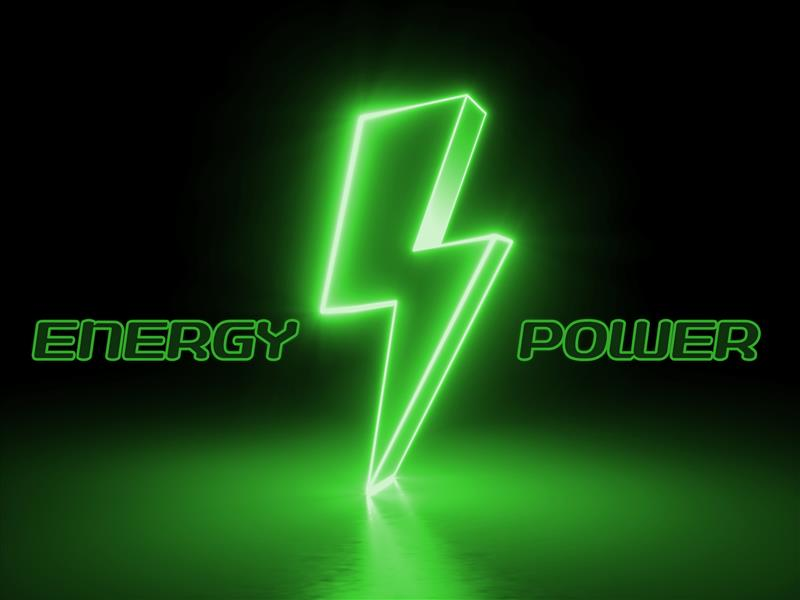# Energy vs. Power – what's the difference?

A helpful guide to telling the difference between power and energy
Watch the full webinar on demandIf you’ve done some research on solar, you have probably seen terms like “power” and “energy”. You may have asked yourself, “What’s the difference between the two? Are they the same thing?” If so, you’re not alone.

Many confuse the definitions between “power” and “energy”. This article will help you understand the difference between the two and how the terms relate to solar.

### What is energy?

Energy is a measurement of the ability of something to do work, and it can be stored and measured in many forms. When it comes to electricity, energy is measured in Watt-hours (Wh), kilowatt-hours(kWh), megawatt-hours (MWh), etc. For example, in households, a common form of electricity and energy consumption is lighting. If you’re using a 60W light for 5 hours, that’s 60W x 5hours = 300Wh of energy required to keep it on.

### What is power?

Power is the rate at which energy is transferred, and it’s typically measured in watts (W), kilowatts (kW), megawatts (MW), etc. Using the light example above, the 60W rating is the power rating of the lightbulb. It’s the rate at which energy is transferred, in this case from electricity to light, over time. If we think about it in reverse, then Power = Energy / Time.

### Analogies of energy and power

Let’s look at some analogies to help solidify your understanding of the two terms.

In the case of driving a car, power is the rate at which you drove (in miles per hour or kilometers per hour), and energy is the total distance you drove (in miles or kilometers).

In the case of a hose with running water going into a bucket, power is the flow rate of the water (liters/second or gallons/second), and energy is how much water ends up in the bucket (liters or gallons).

Too boring? A physics professor had some fun and (with significant assumptions) estimated that a lightsaber requires 28kW of power to operate. So, if Luke needs it for a 10-minute fight, that’s 28kW x (10mins / 60 mins per hour)= 7kWh of energy. If Luke fought for 3 hours, his lightsaber would consume 28kW x 3 hours = 84kWh of energy.

# Energy and power in solar

Now, let’s apply our learnings to solar.  Power (kW) is the rate at which your solar photovoltaic (PV) system generates electricity, and energy (kWh) is the sum of the electricity that is generated over time.

Let’s use a visual example from the Tigo EI Monitoring Portal. This chart shows the power (kW) on the y-axis and time on the x-axis. You can see that the solar installation power production hit a peak of just over 3.0kW at about 2 pm. Then it quickly dropped off from clouds and was variable for the remainder of the day.

The area under the curve is the total energy produced in a given time period. This chart shows the energy produced for each hour of the day, where Energy (kWh) = Average Power (kW) x 1 hour. The 1 O’clock hour shows a total of just over 3 kWh. For this day in May, this solar system produced a total of 21 kWh of energy.

Energy production can also be shown across an entire day, like the example below. A simple way to calculate savings is to multiply the energy produced each day (kWh) x the utility rate (\$/kWh) to get \$ savings. For example, if the system produced 20 kWh in one day and the utility rate was \$0.18/kWh, the savings for that day would be \$3.60.

We can view energy production in many different time formats; for example, below we can see how much energy was produced in each month of 2021.

### Batteries

Battery datasheets will often state energy and power ratings. For example, the Tigo EI Battery has a rating of 10kWh of energy capacity and 5kW of continuous power. Doing the math, we can quickly see that it can supply the maximum continuous output of 5kW to a home for 2 hours (10kWh / 5kW = 2h). This is often referred to as a 2-hour battery.

Battery sizing is a long topic for another discussion, but these two numbers are critical in the following ways:

• The battery power(kW) determines the maximum loads the battery can serve. Think lights +appliances + air conditioner + etc. With more battery power, more loads can be served.
• The battery energy (kWh) is the ability to service those loads over time. A typical refrigerator requires about 300W to operate when the compressor turns on. On average, most refrigerator compressors run about 6 to 8 hours a day which means it will consume about 2 kWh per day of battery capacity.

### Summary

To summarize, energy is the total amount produced or generated, and power is the rate of, or how much, energy is transferred over time.

By understanding the concept of energy and power, you can calculate your savings and determine the energy requirements you need to power your home.

If you have any questions on the subject, feel free to leave us a comment here.

If you want to learn more about solar, follow us on social media to be notified when a new blog is released.

ShareVIEW ALL

VIEW ALL